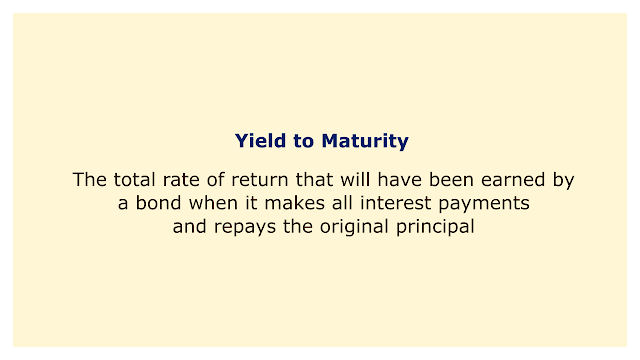# Yield to MaturityImage: Moneybestpal.com

### Yield to maturity (YTM) is the overall rate of return that a bond will have earned once all interest payments are made and the principal is repaid. In essence, YTM represents the internal rate of return on a bond if held to maturity.

A key tenet of YTM is that every coupon or interest payments are reinvested at the same rate of return as the bond, with the investor purchasing the bond at the current market price and holding it until it matures. The temporal value of money is considered by YTM as well as the present value of anticipated cash flows.

The annual interest rate that a bond pays depending on its face value is called the coupon rate. YTM is not the same as the coupon rate. While the YTM fluctuates based on the bond's market price and remaining maturity time, the coupon rate does not.

#### How to Calculate Yield to Maturity?

The formula to calculate the YTM of a bond is as follows:

YTM=nCurrent PriceFace Value1

Where:
• FV = Face value of the bond
• PV = Present value or market price of the bond
• n = Number of years to maturity

The equation demonstrates an inverse relationship between YTM and the bond's market price. Higher YTM results from lower prices and vice versa. This is due to the fact that a lower price indicates a larger necessary return on the bond or discount rate.

The aforementioned formula, however, only works with approximations of YTM. A financial calculator or the trial-and-error method must be used to determine the exact YTM. This is due to the formula's unrealistic assumption that all coupon payments can be reinvested at the same pace as the YTM.

#### Why is Yield to Maturity Important?

Yield to maturity is significant because it enables investors to evaluate bonds of various prices, coupon rates, and maturities on an even playing field. Investors can see which bond delivers the best return based on their investment horizon and risk tolerance by using YTM.

Investors can determine if a bond is overvalued or underpriced based on yield to maturity as well as the bond's intrinsic value. When a bond's yield-to-maturity (YTM) rate exceeds its coupon rate, it is trading below its face value and is expected to generate a bigger return than usual. On the other hand, if a bond's YTM is lower than its coupon rate, it is being sold for more than its face value and will provide a lesser return than anticipated.

However, yield to maturity also has some restrictions. For starters, it does not take into account taxes or other costs that can have an impact on a bond's real yield. Another concern is that it does not account for the possibility of an issuer calling or redeeming a bond prior to maturity, which would diminish the bond's anticipated cash flows. Investors can therefore also consider other bond performance indicators, such as yield to call or yield to worst.
Tags